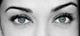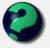# Math Is Fun Forum

Discussion about math, puzzles, games and fun.   Useful symbols: ÷ × ½ √ ∞ ≠ ≤ ≥ ≈ ⇒ ± ∈ Δ θ ∴ ∑ ∫  π  -¹ ² ³ °

You are not logged in.

## #1 2007-01-09 08:32:31

unique
MemberRegistered: 2006-10-04
Posts: 419

### in my view it seems right

use the remainder theorem to evaluate 4x^3 + 2xx^2 + 3 + 1 , when x = -2

4(-2)^3 + 2(-2)^2 + 3(-2) + 1
-32 + 8 + (-6) + 1
-32 + 8 - 6 + 1
-24 - 6 + 1
-30 + 1
-29

Desi
Raat Key Rani !

Offline

## #2 2007-01-09 10:13:11

mathsyperson
ModeratorRegistered: 2005-06-22
Posts: 4,900

### Re: in my view it seems right

I'm assuming there's a typo in your top line and that the 3 should be 3x. If so, then you have the right answer, but are using the wrong method.

To find the answer using the remainder theorem, you need to divide (4x³ + 2x² + 3x + 1) by (x+2). The remainder of that will be the value of the function at x=-2, which as you've already found, is -29.

Why did the vector cross the road?
It wanted to be normal.

Offline